# Introduction to Limits JEE Notes | EduRev

## JEE : Introduction to Limits JEE Notes | EduRev

The document Introduction to Limits JEE Notes | EduRev is a part of the JEE Course Mathematics (Maths) Class 11.
All you need of JEE at this link: JEE

A. Definition of Limit

Let f be a function defined on an open interval containing c (except possibly at c) and let L be a real number. The statement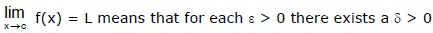such that if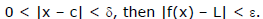B. The Existence of a Limit

Let f be a function and let c and L be real numbers. The limit of f(x) as x approaches c is L if and only if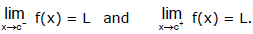In other words limit of a function f(x) is said to exist as, x→a when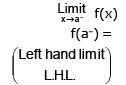=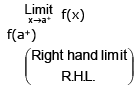=    A finite quantity .

Ex.1 The graph of a function g is shown in the figure. Use it to state the values (if they exist) of the following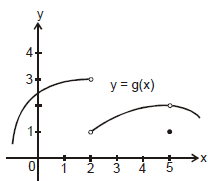(a)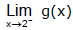(b)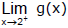(c)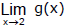(d)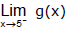(e)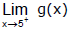(f)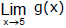Sol. From the graph we see that the values of g(x) approach 3 as x approaches 2 from the left, but they approach 1 as x approaches 2 from the right. Therefore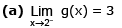and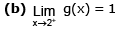(c) Since the left and right limits are different, we conclude that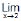g(x) does not exist.

The graph also show that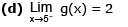and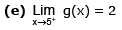(f) This time the left and right limits are the same and so, we have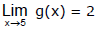Despite this fact, notice that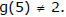Ex.2 If f(x) =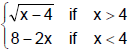determine whether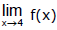exists.

Sol.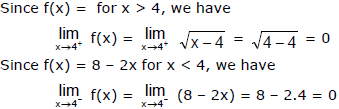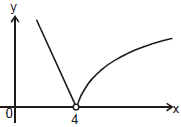The right and left-hand limits are equal. Thus, the limit exists and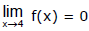The graph of f is shown in the figure.

Ex.3 Evaluate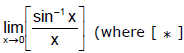denotes the greatest integer function)

Sol.

Let P =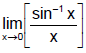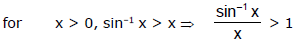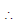R.H.L. =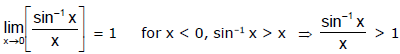∴ L.H.L. =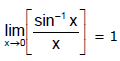Hence P = 1.

C. FUNDAMENTAL THEOREMS ON LIMITS

Let b and c be real numbers, let n be a positive integer, and let f and g be functions with the following limits.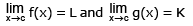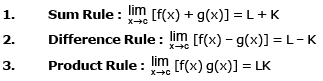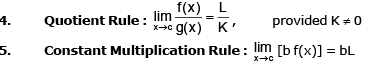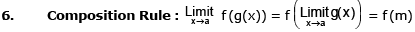provided f is continuous at x = m.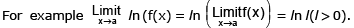Ex.4 Evaluate the following limits and justify each step.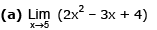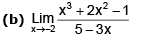Sol.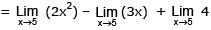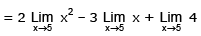= 2(52) – 3(5) + 4 = 39

(b) We start by using laws of limit, but their use is fully justified only at the final stage when we see that the limits of the numerator and denominator exist and the limit of the denominator is not  0.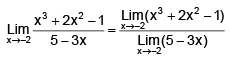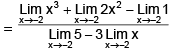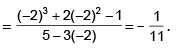D. NON-EXISTENCE OF LIMIT

Three of the most common types of behaviour associated with the non-existence of a limit.

1. f(x) approaches a different number from the right side of c than it approaches from the left side.

2. f(x) increases or decreases without bound as x approaches c.

3. f(x) oscillates between two fixed values as x approaches c.

There are many other interesting functions that have unusual limit behaviour. An often cited one is the Dirichlet function f(x)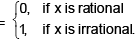This function has no limit at any real number c.

E. INDETERMINANT  FORMS :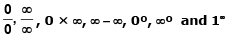Ex.5 Which of the following limits are in indeterminant forms. Also indicate the form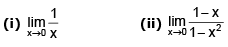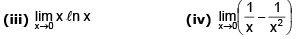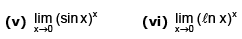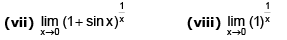Sol. (i) No (ii) Yes 0/0 form (iii) Yes 0 × ∝ form (iv) Yes (∝ – ∝) form

(v) Yes, 00 form (vi) Yes ∝0 form (vii) Yes 1∝form (viii) No

Remark :

(i)   ‘0’ doesn’t means exact zero but represent a value approaching towards zero similarly to ‘1’ and infinity.

(ii) ∝+ ∝= ∝(iii) ∝× ∝= ∝(iv) (a/) = 0 if a is finite

(v) a/0 is not defined for any a ε R.

(vi)  a b = 0, if & only if a = 0 or b = 0 and a & b are finite.

Offer running on EduRev: Apply code STAYHOME200 to get INR 200 off on our premium plan EduRev Infinity!

## Mathematics (Maths) Class 11

158 videos|179 docs|161 tests

,

,

,

,

,

,

,

,

,

,

,

,

,

,

,

,

,

,

,

,

,

;